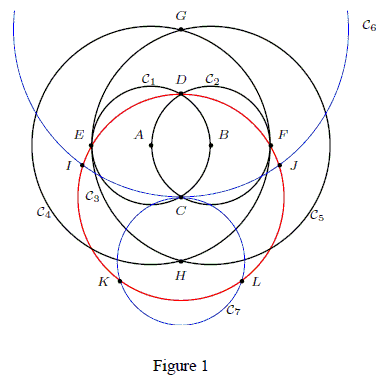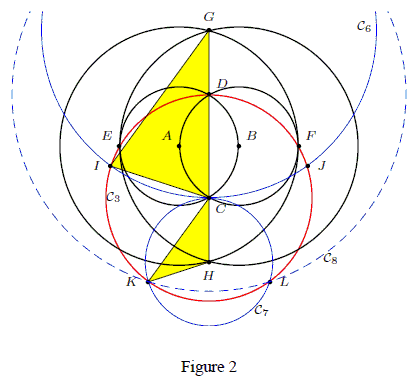Mascheroni Construction of a Regular Pentagon

What follows is a reproduction of a short note by Kurt Hofstetter giving a compass-only construction of a regular pentagon:

In 2002 we have given a simple 5-step compass-only (Mascheroni) construction of the golden section. Here we note that with two additional circles, it is possible to construct the vertices of a regular pentagon. As usual, we denote by P(Q) the circle with center P and passing through Q.Construction 1. Given two points A and B,

1. C1 = A(B),
2. C2 = B(A) to intersect C1 at C and D,
3. C3 = C(D) to intersect C1 at E and C2 at F,
4. C4 = A(F),
5. C5 = B(E) to intersect C4 at G and H.
6. C6 = G(C) to intersect C3 at I and J,
7. C7 = H(C) to intersect C3 at K and L.

Then DIKLJ is a regular pentagon.

Proof: In a 2002 paper, we have shown that the first five steps above lead to four collinear points C, D, G, H such that D divides CG, and C divides DH, in the golden section.1. This means that in the isosceles triangle GCI, GC/IC = GC/DC = φ.. The base angles are 72° Therefore, ∠DCI = 72° By symmetry, ∠DCJ = 72°.

2. Also, in the isosceles triangle HCK, KC/CH = DC/CH = φ.. The base angles are ∠36°. It follows that ∠KCH = 36° By symmetry, ∠LCH = 36°, and ∠KCL = 72°.

3. Since C is on the line GH, ∠ICK = 180° - ∠GCI - &anf;KCH = 72°. By symmetry, ∠JCL = 72°.

Therefore, the five points D, I, K, L, J are equally spaced on the circle C3.

They form the vertices of a regular pentagon.

Remark. The circle C7 can be replaced by C8 with center D and radius IJ. This intersects C3 at the same points K and L.

References

1. K. Hofstetter, A Simple Compass-Only Construction of the Regular Pentagon, Forum Geometricorum Volume 8 (2008) 147-148.